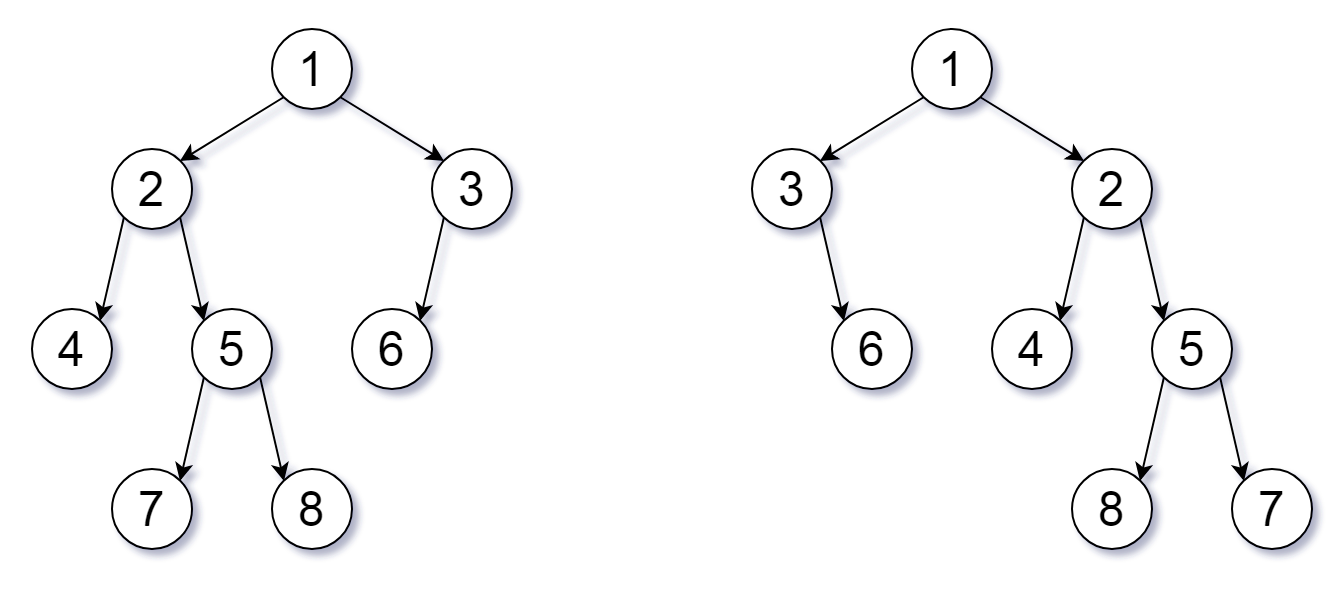@Yano 2019-09-20T02:48:22.000000Z 字数 1063 阅读 1167

# LeetCode 951 Flip Equivalent Binary Trees

LeetCode

# 公众号

coding 笔记、点滴记录，以后的文章也会同步到公众号（Coding Insight）中，希望大家关注^_^

https://github.com/LjyYano/Thinking_in_Java_MindMapping# 题目描述

For a binary tree T, we can define a flip operation as follows: choose any node, and swap the left and right child subtrees.

A binary tree X is flip equivalent to a binary tree Y if and only if we can make X equal to Y after some number of flip operations.

Write a function that determines whether two binary trees are flip equivalent. The trees are given by root nodes root1 and root2.

Example 1:

Input: root1 = [1,2,3,4,5,6,null,null,null,7,8], root2 = [1,3,2,null,6,4,5,null,null,null,null,8,7]
Output: true
Explanation: We flipped at nodes with values 1, 3, and 5.
Flipped Trees DiagramNote:

Each tree will have at most 100 nodes.
Each value in each tree will be a unique integer in the range [0, 99].


# 代码

public boolean flipEquiv(TreeNode root1, TreeNode root2) {    if (root1 == null && root2 == null) return true;    if (root1 == null || root2 == null) return false;    if (root1.val != root2.val) {        return false;    }    return (flipEquiv(root1.left, root2.left) && flipEquiv(root1.right, root2.right)) ||            (flipEquiv(root1.left, root2.right) && flipEquiv(root1.right, root2.left));}• 私有
• 公开
• 删除